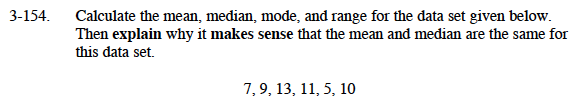### Home > MC1 > Chapter 3 > Lesson 3.4.5 > Problem3-154

3-154.

.Calculate the mean, median, mode, and range for the data set given below. Homework Help ✎
Then explain why it makes sense that the mean and median are the same for this data set.
7, 9, 13, 11, 5, 10Refer to problem 3-85 if you cannot remember what mean, median, and mode are.
The range is the difference between the largest number and the smallest number in the data.

Since the mean is an average, does it make sense that it would be very similar to the median?